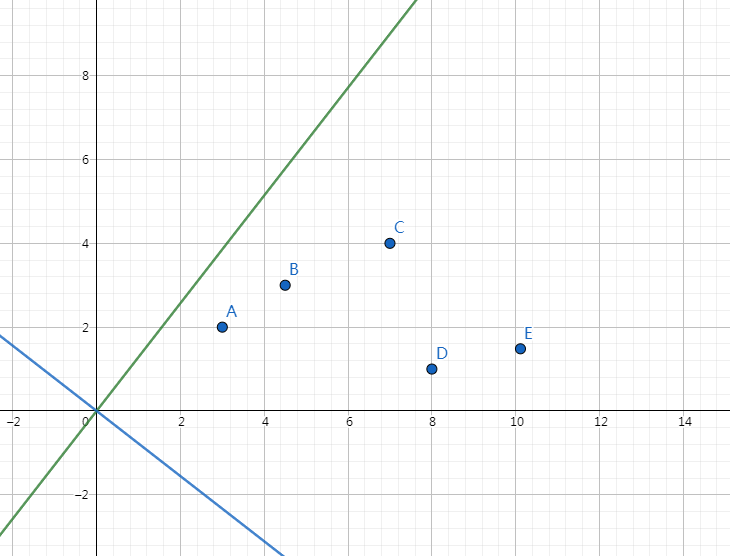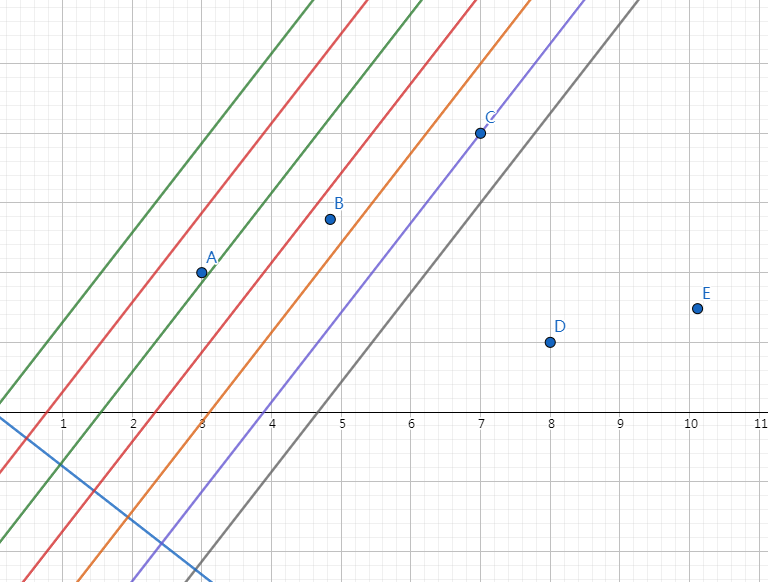$std$ 给出的做法是每个点以过它本身的斜率为 $\frac{P}{Q}$ 的直线的截距排序，然后从排序后相邻的点对中计算最优解，正确性的证明与上述过程基本一致。复杂度 $O(N log N)$

#include<stdio.h>
#include<string.h>
#include<algorithm>
using namespace std;

x=0; char c=getchar();
while(c<'0'||'9'<c)c=getchar();
while('0'<=c&&c<='9'){x=(x<<1)+(x<<3)+(c^48); c=getchar();}
}
typedef long long ll;
typedef long double db;
const int N=200050;
const db e=1e-15;
int n;
ll P,Q,p,q;
struct point{ll x,y,c;}a[N];
ll gcd(ll _a,ll _b){return _b==0?_a:gcd(_b,_a%_b);}
ll absl(ll _a){return _a>0?_a:-_a;}
db absd(db _a){return _a>0?_a:-_a;}
bool cmp(point x,point y){return x.c<y.c;}

int main(){
freopen("slope.in","r",stdin);
freopen("slope.out","w",stdout);
ll d=gcd(P,Q); P/=d; Q/=d;
for(int i=1,x,y;i<=n;i++){
a[i]=(point){x,y,1ll*Q*y-1ll*P*x};
}
sort(a+1,a+n+1,cmp);
ll tp,tq,p,q;
db r=1e10,t,s=(db)P/(db)Q;
for(int i=1;i<n;i++){
tp=a[i].y-a[i+1].y;
tq=a[i].x-a[i+1].x;
if(tp*tq<0) continue;
tp=absl(tp); tq=absl(tq);
d=gcd(tp,tq); tp/=d; tq/=d;
t=absd((db)tp/(db)tq-s);
if(r-t>e) r=t, p=tp, q=tq;
}
printf("%lld/%lld\n",p,q);
return 0;
}

#include<stdio.h>
#include<string.h>
#include<algorithm>
using namespace std;

x=0; bool f=0; char c=getchar();
while(c<'0'||'9'<c){f|=(c=='-'); c=getchar();}
while('0'<=c&&c<='9'){x=(x<<1)+(x<<3)+(c^48); c=getchar();}
x=f?-x:x;
}
typedef long long ll;
const int N=200050;
int n,A,B,C,D;
int a[N],top;
struct node{int i; ll v;}st[N];
ll f[N];

ll F(int x){return 1ll*A*x*x*x+1ll*B*x*x+1ll*C*x+D;}

int main(){
freopen("min.in","r",stdin);
freopen("min.out","w",stdout);
ll tmp=0;
for(int i=1;i<=n;i++){
tmp=f[i-1];
while(top&&a[st[top].i]>a[i]) tmp=max(tmp,st[top--].v);
f[i]=tmp+F(a[i]);
if(top) f[i]=max(f[i],f[st[top].i]);
st[++top]=(node){i,tmp};
}
printf("%lld\n",f[n]);
return 0;
}